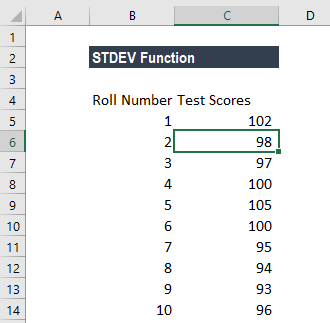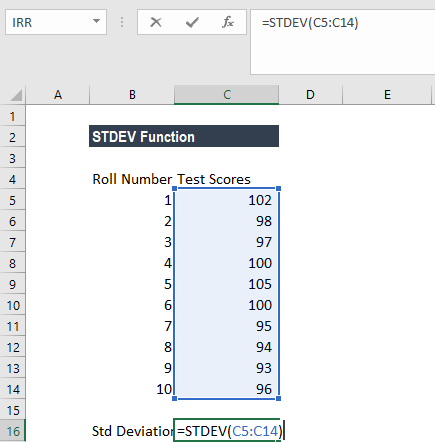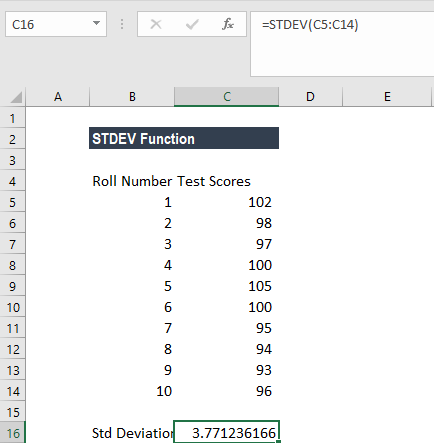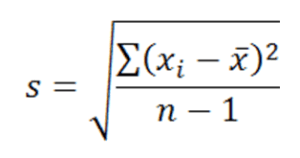# STDEV Function

Estimates the standard deviation based on a sample

## What is the STDEV Function?

The STDEV Function is categorized under Excel Statistical functions. The function will estimate the standard deviation based on a sample.

As a financial analyst, STDEV can be useful in using the annual rate of return on an investment to measure its volatility. A risky stock will show a higher standard deviation while a safer stock, such as blue-chip companies like Apple or Coca-Cola, will show a lower standard deviation.

### Formula

=STDEV(number1,[number2],…)

The STDEV function uses the following arguments:

1. Number1 (required argument) – This is the first number argument that corresponds to a sample of a population.
2. Number2 (optional argument) – This is a number argument that corresponds to a sample of a population.

#### Notes

1. Arguments must provide two or more numeric values to the function.
2. The function can contain 1–255 numeric arguments.
3. Arguments can either be numbers, arrays, or references that contain numbers.
4. STDEV is used to calculate the standard deviation of a population sample. To calculate the standard deviation of a whole population, we should use STDEVP or STDEV.P instead.

### How to use the STDEV Function in Excel?

As a worksheet function, STDEV can be entered as part of a formula in a cell of a worksheet. To understand the uses of the function, let us consider an example:

#### Example

Suppose we are given the test scores of students below:For the standard deviation, the formula used is:We get the results below:The equation used is:Where:

• takes on each value in the set
• is the average (statistical mean) of the set of values
• is the number of values

### Things to remember about the STDEV Function:

1. #DIV/0! error – Occurs if less than two numeric values are provided to the STDEV function.
2. #VALUE error – Happens if any of the given values to the function are text values.
3. In MS Excel 2010, the STDEV function has been updated to the STDEV.S function. However, STDEV can still be used to allow for backward compatibility with older versions of Excel.

Thanks for reading CFI’s guide to important Excel functions! By taking the time to learn and master these functions, you’ll significantly improve your financial modeling in Excel. To learn more, check out these additional CFI resources:

• Excel Functions for Finance
• All Excel Functions
• Financial Modeling Best Practices
• Financial Analyst Certification

### Free Excel Tutorial

To master the art of Excel, check out CFI’s FREE Excel Crash Course, which teaches you how to become an Excel power user.  Learn the most important formulas, functions, and shortcuts to become confident in your financial analysis.

Launch CFI’s Free Excel Course now to take your career to the next level and move up the ladder!